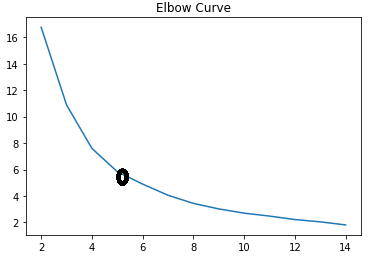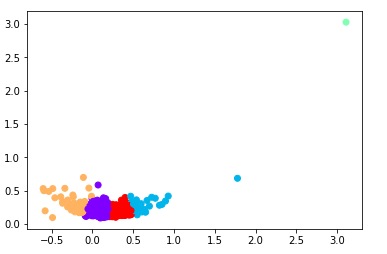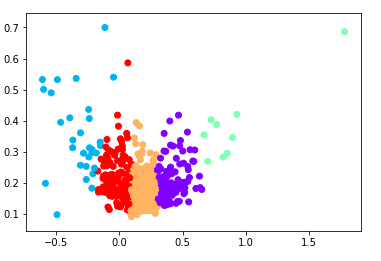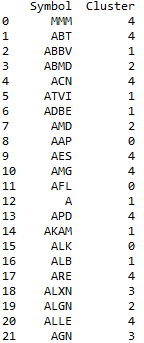# K-Means Algorithm Python Example

In this post, we will provide an example of the implementation of the K-Means algorithm in python.  This K-Means algorithm python example consists of clustering a dataset that contains information of all the stocks that compose the Standard & Poor Index.

This example contains the following five steps:

• Obtain the 500 tickers for the SPY & 500 by scrapping the tickers symbols from Wikipedia. The function obtain_parse_wike_snp500() conduct this task.
• Obtain closes prices from last year for each of the symbols using the Quandl API
• Calculate mean and variance of the returns for each stock
• Choose the best k value for the cluster the dataset
• Fit the model with the k number of cluster
import pandas as pd
import numpy as np
from math import ceil
import bs4
import requests
import quandl # need to do pip install quandl
from sklearn.cluster import KMeans
import matplotlib.pyplot as plt

def obtain_parse_wiki_snp500():
""" Download and parse the Wikipedia list of S&P500 constituents using requests and Beautiful Soup.
"""

response = requests.get( "http://en.wikipedia.org/wiki/List_of_S%26P_500_companies" )

soup = bs4.BeautifulSoup(response.text)

# This selects the first table, using CSS Selector syntax and then ignores the header row ([1:])
symbolslist = soup.select('table').select('tr')[1:]

# Obtain the symbol information for each row in the S&P500 constituent table
symbols = []
for i, symbol in enumerate(symbolslist):
tds = symbol.select('td')
symbols.append( (tds.select('a').text, # Ticker
tds.select('a').text, # Name
tds.text # Sector
)
)

return symbols

tickers = obtain_parse_wiki_snp500()


The tickers object is a list of tuples with the ticker, company name and sector of each company. To observe the structure of the tickers object we show the first 10 elements.

tickers[:10]

[('MMM', '3M Company', 'Industrials'),
('ABT', 'Abbott Laboratories', 'Health Care'),
('ABBV', 'AbbVie Inc.', 'Health Care'),
('ABMD', 'ABIOMED Inc', 'Health Care'),
('ACN', 'Accenture plc', 'Information Technology'),
('ATVI', 'Activision Blizzard', 'Communication Services'),
('AMD', 'Advanced Micro Devices Inc', 'Information Technology'),
('AAP', 'Advance Auto Parts', 'Consumer Discretionary'),
('AES', 'AES Corp', 'Utilities')]


We will use this list in the following function to loop over each symbol of tickers and get the close price from the Quandl API. We perform the loop using a try-except block to handle errors regarding symbols that were changed or are different in the Quandl database.

def get_quandl_data(symbols):
"""
This function would loop over all the symbols from the SPY & 500 and retrieve the Close price column  from the WIKI database from Quandl between the dates given by the start_date and end_date parameters
"""

symbols = [symbol for symbol in symbols]
stocks_info = []

for symbol in symbols:
try:
stockdata = quandl.get("WIKI/{}".format(symbol), start_date='2018-09-20',       end_date='2019-09-20',column_index=4)
stocks_info.append((symbol,stockdata.iloc[:,0]))
except:
print('The stock symbol {} is not on quandl database'.format(symbol))

return stocks_info

data = get_quandl_data(tickers)


The data object is a list of tuples where each tuple has 2 elements. The first element is the ticker and the second element is the Date and Close Price for each of the stocks. We need to parse this information in order to make a dataframe that has the date as index and the close prices for each stock as columns. The following lines will do this job:

# Get only the symbols from the data object
symbols = [data[i] for i in range(0,len(data))]
# Get the date and prices from the data object (second element of the tuple)
closes = [data[i] for i in range(0,len(data))]

# Store closes object  in a dataframe and obtain the transpose of the dataframe. #With this we will have the Date as index and the Prices of each stock as columns.
closes = pd.DataFrame(closes).T
# Rename column names with the symbols list
closes.columns = symbols
#Calculating annual mean returns and standard deviation of the returns
returns = closes.pct_change().mean() * 252
std = closes.pct_change().std() * np.sqrt(252)

#Concatenating the returns and variances into a single data-frame
ret_var = pd.concat([returns, std], axis = 1).dropna()
ret_var.columns = ["Returns","Standard Deviation"]


The ret_var dataframe has the following structure:

ret_var.head(10)

Returns              Standard Deviation
MMM           0.301229                   0.122294
ABT               0.392332                    0.138839
ABBV            0.457778                    0.179314
ABMD          0.545361                    0.242094
ACN              0.284499                    0.131696
ATVI             0.596721                    0.305587
AMD            0.069001                     0.585976
AAP             -0.460970                    0.394637
AES              -0.052332                    0.210608


In order to determine the optimal number of clusters k for the ret_var dataset, we will fit different models of the K-means algorithm while varying the k parameter in the range 2 to 14. For each model we calculate the Sum Squared Error (SSE) by using the _inertia__ method of the model fitted. In each iteration we append the inertia to the sse list. Then we take the model with the less value of SSE. (Inertia tells how far away the points within a cluster are. The small the inertia value is better.)

#Converting ret_var into numpy array
X =  ret_var.values
sse = []
for k in range(2,15):
kmeans = KMeans(n_clusters = k)
kmeans.fit(X)

#SSE for each n_clusters
sse.append(kmeans.inertia_)

plt.plot(range(2,15), sse)
plt.title("Elbow Curve")
plt.show()


The graph is names as Elbow Curve, and shows that the optimal value of k is 5.

Elbow Curve: Determine the optimal value of k. K-means ClusterWe chose k=5 and fit the model with this parameter-value.

kmeans = KMeans(n_clusters = 5).fit(X)
centroids = kmeans.cluster_centers_
plt.scatter(X[:,0],X[:,1], c = kmeans.labels_, cmap ="rainbow")
plt.show()


The different groups or cluster of the dataset are reflected in the following graph:

Clusters of the ret_var dataset. k=5We can view the presence of outliers as only one point is on the upper right side of the graph. This outlier form its own cluster. In order to have a better categorization of the stocks within the SPY index, we would remove those stocks and fit the model another time.

# Find the stock with the highest value in the Standard Deviation variable
stdOrder = ret_var.sort_values('Standard Deviation',ascending=False)
first_symbol = stdOrder.index

# Drop the columns with the outliers values
ret_var.drop(first_symbol,inplace=True)
# Fit the model without the outliers
X = ret_var.values
kmeans =KMeans(n_clusters = 5).fit(X)
centroids = kmeans.cluster_centers_
plt.scatter(X[:,0],X[:,1], c = kmeans.labels_, cmap ="rainbow")
plt.show()


Clusters of ret_var dataset without Outliers. k=5The x axis of the Figure 17, refers to the returns of the stocks and the y axis is the standard deviation of each stock. So the stocks that are in the upper-right cluster are the stocks with the higher value of returns and standard deviation.

Finally we will assign to each stock it correspondent number of cluster(1,2,3,4,and 5) and make a dataframe with this information. Having the information of cluster number for each stock, we can create a diversified portfolio in the long term, between stocks from different clusters.

stocks = pd.DataFrame(ret_var.index) # the dataframe structure allow concatenation
cluster_labels = pd.DataFrame(kmeans.labels_)
stockClusters = pd.concat([stocks, cluster_labels],axis = 1)
stockClusters.columns = ['Symbol','Cluster']


The structure of the stockClusters dataframe is the following:These are the first rows of the stockClusters dataframe. We conclude this section with a categorization of each stock from the SPY & 500 in terms of returns and risk. This could be an important tool for portfolio diversification. This concludes our K-Means algorithm python example.

Get smart about tech at work.

As a non-technical professional, learn how software works with simple explanations of tech concepts. Learn more...

# Data Science for Finance Bundle: 43% OFF

Get our Data Science for Finance Bundle for just $29$51.